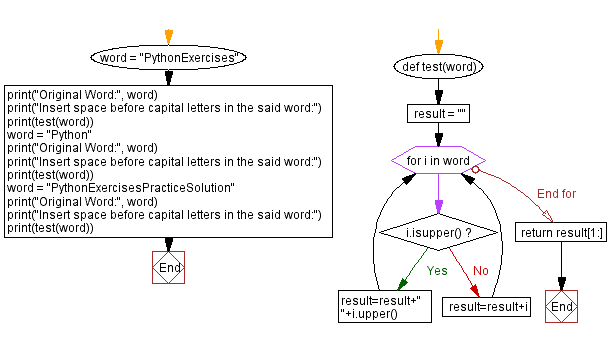﻿ Python - Insert space before capital letters in word# Python Exercises: Insert space before capital letters in word

## Python String: Exercise-110 with Solution

Write a Python program to insert space before every capital letter appears in a given word.

Sample Data:
("PythonExercises") -> "Python Exercises"
("Python") -> "Python"
(“PythonExercisesPracticeSolution”) -> “Python Exercises Practice Solution”

Sample Solution:

Python Code:

``````def test(word):
result = ""
for i in word:
if i.isupper():
result=result+" "+i.upper()
else:
result=result+i
return result[1:]

word = "PythonExercises"
print("Original Word:", word)
print("Insert space before capital letters in the said word:")
print(test(word))
word = "Python"
print("Original Word:", word)
print("Insert space before capital letters in the said word:")
print(test(word))
word = "PythonExercisesPracticeSolution"
print("Original Word:", word)
print("Insert space before capital letters in the said word:")
print(test(word))
```
```

Sample Output:

```Original Word: PythonExercises
Insert space before capital letters in the said word:
Python Exercises
Original Word: Python
Insert space before capital letters in the said word:
Python
Original Word: PythonExercisesPracticeSolution
Insert space before capital letters in the said word:
Python Exercises Practice Solution
```

Flowchart:## Visualize Python code execution:

The following tool visualize what the computer is doing step-by-step as it executes the said program:

Python Code Editor:

Have another way to solve this solution? Contribute your code (and comments) through Disqus.

Previous Python Exercise: Count the number of leap years within the range.
Next Python Exercise: Alphabet position in a string.

What is the difficulty level of this exercise?

Test your Programming skills with w3resource's quiz.

﻿

## Python: Tips of the Day

Getting sorted iterable (can sort by "compare" function):

```>>> a = [1, 2, -3]
>>> sorted(a)
[-3, 1, 2]

>>> sorted(a,key=abs)
[1, 2, -3]
```